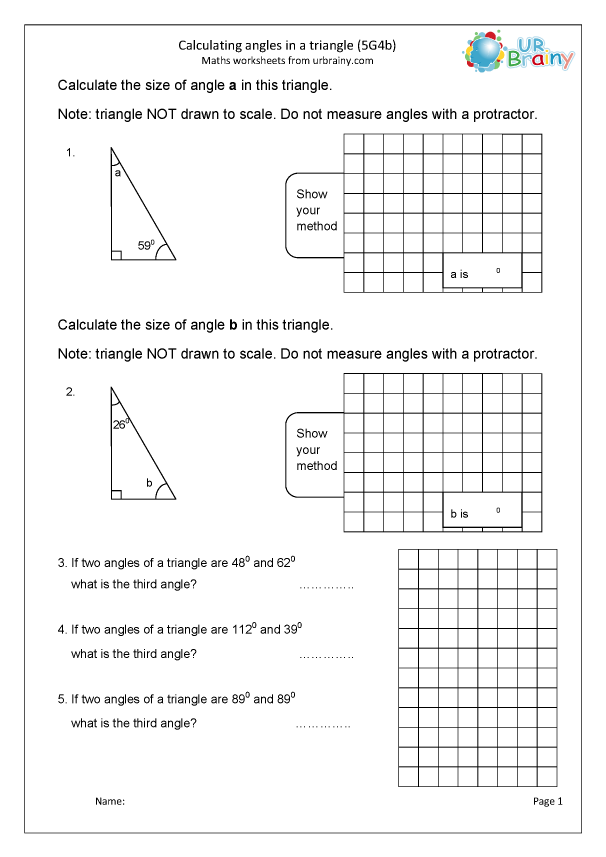## Calculate angles in a triangle (5G4b)

Calculating angles in a triangle: note that these are not drawn to scale so the angle cannot be measured, it has to be calculated from the knowledge that there are 180 degrees. in a triangle.including a 7 day free trial of everything

• View / Print monthly free resources forever
• View everything for 7 days